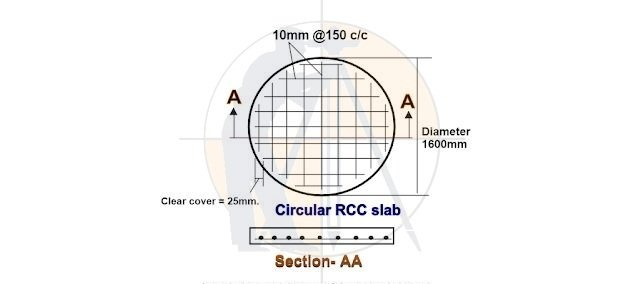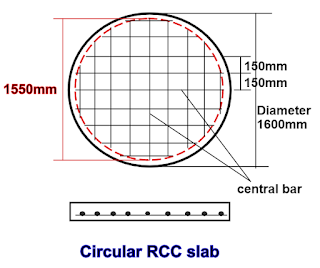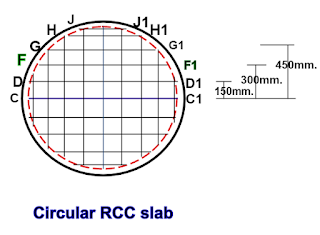Breaking News
Home / Civil Engineering / Bar Bending Schedule / How to calculate the cutting length of reinforcement bars in circular R.C.C slab

# How to calculate the cutting length of reinforcement bars in circular R.C.C slab

### How to calculate the cutting length of reinforcement bars in circular R.C.C slab

Now, let us go through the procedure to find out the cutting length of the bars in a circular slab as shown below.### Given data :

Diameter of the circular slab = 1600mm = 1.6m.

Clear cover for the reinforcement = 25mm.

Rebar diameter = 10mm.

Rebar spacing = 150mm c/c.

The formula for finding the length of the bar

=2√r2 – d2

### Where,

r = [diameter of the slab – (2nos.× clear cover )] ÷ 2

d = c/c distance of the individual bars from the central bar of the circle.As you can observe in the above-given drawing, I have drawn a red-colored circle by deducting the clear cover. Radius r in the above formula will be the radius of this red circle.

i.e. r = [1600mm – ( 2nos.× 25mm)] ÷ 2
= [1550mm ] ÷ 2

### = 775mm.

The value of r will be the same for the cutting length calculation of every individual bar of the slab.

### Note: All the rebars of the circular slab act as a chord of the red circle. To gain a basic understanding of this theory, you have to go through the article,The length of the individual bars in the upper half part of the circular slab will be different.
The cutting length of the bars in the lower half portion will be equal to the upper half part bars.
I have named the bars for the half portion of the Circular slab, and let us find out the cutting length of each of these bars.

### 2. Cutting length of bar DD1

=2√r2 – d2

Here, d = 150mm.

= 2 × √7752 – 1502

= 2 × √ 600625 – 22500

= 2 × √ 578125

= 2 × 760.345

= 1520.69mm = 1.521m.

### 3. Cutting length of the bar FF1

Here, d = 300mm.

=2√r2 – d2

= 2 × √7752 – 3002

= 2 × √ 600625 – 90000

= 2 × √ 510625

= 2 × 714.58

= 1429.16mm = 1.429m.

### 4. Cutting length of the bar GG1

Here, d = 450mm.

=2√r2 – d2

= 2 × √7752 – 4502

= 2 × √ 600625 – 202500

= 2 × √ 398125

= 2 × 630.971

= 1261.94mm = 1.261m.

### 5.Cutting length of the bar HH1

Here, d = 600mm.

=2√r2 – d2

= 2 × √7752 – 6002

= 2 × √ 600625 – 360000

= 2 × √ 240625

= 2 × 490.535

= 981.07mm = 0.981m.

### 6. Cutting length of the bar JJ1

Here, d = 750mm.

=2√r2 – d2

= 2 × √7752 – 7502

= 2 × √ 600625 – 562500

= 2 × √ 38125

= 2 × 195.256

= 390.51mm = 0.39 m

3. ### Basic Components of Building Structure | Building Elements

The calculation should be done until the cumulative value of d is less than r. So, we have calculated the cutting length of 5 bars on the upper side of the central bar CC1.

### No. of bars in a circular slab

The no. of top bars in  the circular slab
=[( diameter of the slab) ÷ c/c bar spacing ] +1

= [ (  1600mm ) ÷  150mm] +1

= 11 nos.
That means 5 bars on either side of the central bar CC1

### Total no. of bars

= Top bars + Bottom bars
= 11nos + 11nos.=22nos.

### Estimating Of Steel Bar Quantity for Construction

As the upper half part of the circle is identical to the bottom half part, you will have 2nos of central common bars and 4 nos of the chord bars having the same cutting length.

1. Cutting length of central bar CC1 = 1.55m 👉 2nos.

2. Cutting length of bar DD1           = 1.521m 👉 4nos.

3. Cutting length of bar FF1            = 1.429m 👉 4nos.

4. Cutting length of bar GG1           = 1.261m 👉 4nos.

5. Cutting length of bar HH1           = 0.981m 👉 4nos.

6. Cutting length of bar JJ1             = 0.390m 👉 4nos.

————–

### THANKS.